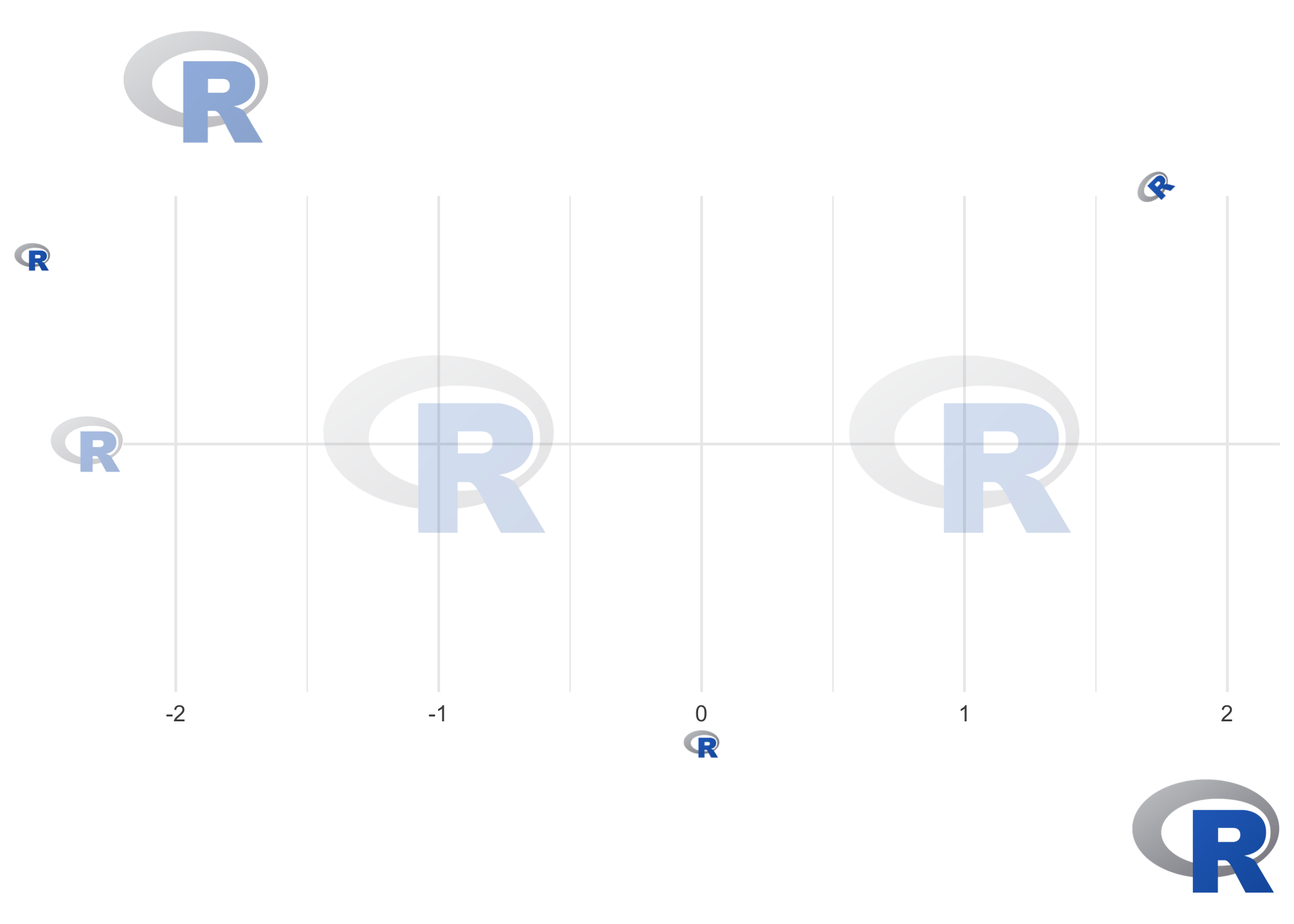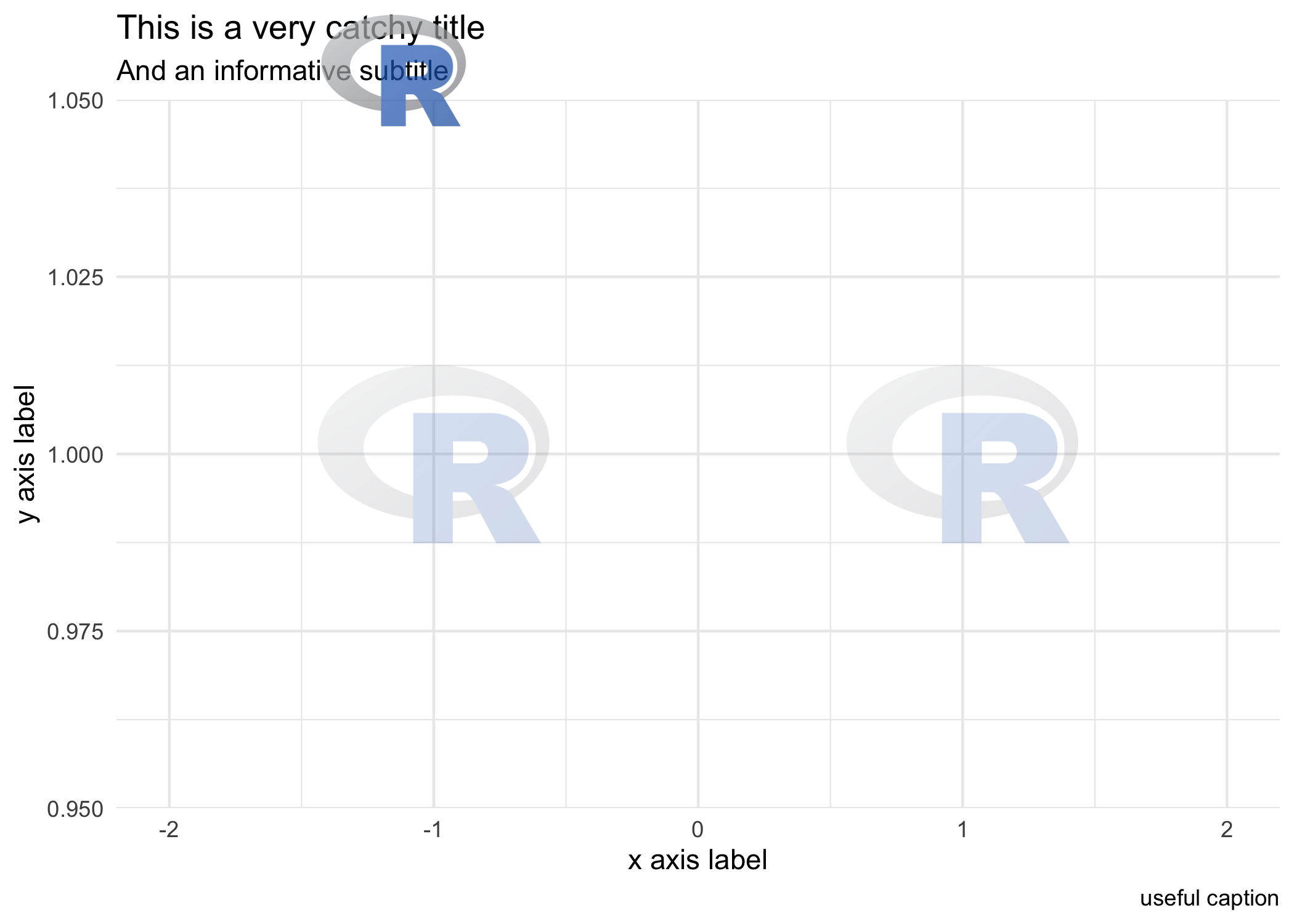ggpath is a ‘ggplot2’ extension that enables robust image grobs in panels and theme elements. This means it helps plotting images (from local paths, from urls or from raw image data) in nearly every part of a ggplot.

## Installation

The easiest way to get ggpath is to install it from CRAN with:

install.packages("ggpath")

To get a bug fix or to use a feature from the development version, you can install the development version of ggpath from GitHub, for example with:

if (!require("pak")) install.packages("pak")
pak::pak("mrcaseb/ggpath")

## Examples

The two main features to provide images in a ggplot are a geom (geom_from_path) and a theme element (element_path). Both replace image urls, local image paths, or raw image data with the actual image. And to improve performance, the images are cached locally.

The below examples use local image files that are shipped with the package. Let’s locate the images first.

local_image_path <- system.file("r_logo.png", package = "ggpath")

Now, we can make a simple plot, where we use the image like a point by replacing the local path with the actual image.

library(ggplot2)
library(ggpath)
plot_data <- data.frame(x = c(-1, 1), y = 1, path = local_image_path)
ggplot(plot_data, aes(x = x, y = y)) +
geom_from_path(aes(path = path), width = 0.2) +
coord_cartesian(xlim = c(-2, 2)) +
theme_minimal()We can build on top of that by adding new axis labels, axis titles, plot title and subtitle, or a caption and using the ggpath theme element. Note the usage of transparency with the alpha argument, the justification with the hjust/vjust arguments, or the rotation with the angle argument.

ggplot(plot_data, aes(x = x, y = local_image_path)) +
geom_from_path(aes(path = path), width = 0.2, alpha = 0.2) +
coord_cartesian(xlim = c(-2, 2)) +
theme_minimal() +
labs(
title = local_image_path,
subtitle = local_image_path,
x = local_image_path,
y = local_image_path,
caption = local_image_path
) +
theme(
plot.caption = element_path(hjust = 1, size = 0.6),
axis.text.y = element_path(size = 1),
axis.title.x = element_path(),
axis.title.y = element_path(vjust = 0.9),
plot.title = element_path(hjust = 0, size = 2, alpha = 0.5),
plot.subtitle = element_path(hjust = 0.9, angle = 45),
)A popular way to personalize a plot is to include a logo in the title area. As shown above, we can replace the title or subtitle with an image but not combine it with text. So if we want a title, a subtitle and still a logo in the title area, we can use the ggplot2 tag, which is actually used to implement figure numbering.

ggplot(plot_data, aes(x = x, y = 1)) +
geom_from_path(aes(path = path), width = 0.2, alpha = 0.2) +
coord_cartesian(xlim = c(-2, 2)) +
theme_minimal() +
labs(
title = "This is a very catchy title",
subtitle = "And an informative subtitle",
x = "x axis label",
y = "y axis label",
caption = "useful caption",
tag = local_image_path
) +
theme(
plot.tag = element_path(size = 2, vjust = 1, alpha = 0.7),
plot.tag.position = c(0.3,1),
)Please note how to place the image in the whole plot area via plot.tag.position. So in combination with alpha you can place a logo also behind title and subtitle.

## ggpath Options

The option "ggpath.cache" can be used to configure the package cache. It can be set with

options(ggpath.cache = "memory")
# or
options(ggpath.cache = "filesystem")
# or
options(ggpath.cache = "off")

The default - "memory" - caches in the current session, while "filesystem" caches on disk which means that the cache is available after starting a fresh session. All cache options time out after 24 hours.

## Comparison with Similar Image-Plotting Packages

There are various ggplot2 extensions that provide similar functionality in terms of plotting images. These include but not limited to

ggpath combines the strengths of all of the above by providing

• functions to plot images in both the panel (with geom_from_path) and all other plot areas (with element_path),
• robust image aspect ratio,
• options for changing the color of images including a grayscale transformation,
• options for applying transparency, and
• improved performance through image caching.

There are some downsides compared to the above mentioned packages, e.g.